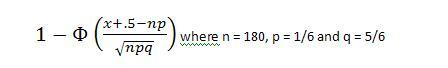# The Normal Distribution and Discrete Populations

• Shoney45

## Homework Statement

One sixth of the male freshman entering a large state school are out of state students. If the students are assigned at random to the formitories, 180 to a building.

1) What approximately is the probability that in a given dormitory at least one fifth of the students are from out of state? (Hint Continuity Correction)

2) What approximately, is the median of the number of the out of state students in a given dormitory?

## Homework Equations## The Attempt at a Solution

The part I am unsure of has to do with the 'continuity correction'. My book gives the impression on the page prior to introducing this formula that continuity correction has to do with subtracting .5 from the value of x.

The explanation for this comes from a section titled 'The Normal Distribution and Discrete Populations'. It says that because the rectangles of a histogram are centered at integers, the rectangle representing 36 actually begins at 35.5. So I am unsure if I should plug in 36, or 35.5 into the formula.

Last edited:
A binomial distribution is discrete while the normal distribution is continuous.

So say you wanted to find P(X≥1), you can use the distribution and find. If you approximated it with the normal, then values more than 0.5 you call as 1.

So 0.6 can be approximated to 1, 0.7 and so forth.

So to use the normal distribution, you'll want to find P(X>0.5) and then apply the transform Z=(X-μ)/σ.

Does it make a little more sense now?

So in your question you want P(X≥36), if you want to use the normal distribution, what probability do you want P(?)

...to use the normal distribution, you'll want to find P(X>0.5) and then apply the transform Z=(X-μ)/σ.

So in your question you want P(X≥36), if you want to use the normal distribution, what probability do you want P(?)

Does it make a little more sense now?

Okay, so since I am looking for P(X≥36), and since that rectangle on the histogram goes from 35.5 - 36.5, then I think you're saying that I need to Z=[(X-μ)/σ] = (35.5 - μ)/σ.

Now I am a little unclear again. Since I am looking for P(X≥36), wouldn't my equation be 1- (35.5 - μ)/σ.

Now I am a little unclear again. Since I am looking for P(X≥36), wouldn't my equation be 1- (35.5 - μ)/σ.

You want 1-P(Z>(35.5 - μ)/σ)

You'll need to calculate both μ and σ.

Remember when you were treating it as a binomial, X~Bin(n,p)

Now you are treating as an approximate normal X≈N(μ,σ2)

So for your binomial, what is E(X) and Var(X) ?

You want 1-P(Z>(35.5 - μ)/σ)

You'll need to calculate both μ and σ.

Remember when you were treating it as a binomial, X~Bin(n,p)

Now you are treating as an approximate normal X≈N(μ,σ2)

So for your binomial, what is E(X) and Var(X) ?

So: μ = np = 180*1/6 = 30 and σ = $$\sqrt{}np(1-p)$$ = 180 * 1/6 * 5/6 = 25

So that the whole thing is 1 - (35.5-30)/25 = 4.5/25 = 1-0.18 = 1-0.5714 (from the table for standard normal curve areas) = 0.4286.

Am I tracking with this now?

I'm also totally stumped on

2) What approximately, is the median of the number of the out of state students in a given dormitory?

What I'm guessing is that I have to set something equal to teh 50th percentile,a nd then use that to approximate the number of out of state students in a dormitory. I just can't figure out how to do it.

I'm also totally stumped on

2) What approximately, is the median of the number of the out of state students in a given dormitory?

What I'm guessing is that I have to set something equal to teh 50th percentile,a nd then use that to approximate the number of out of state students in a dormitory. I just can't figure out how to do it.

In a normal distribution, the mean = mode = median.

Okay, so its that easy eh? So μ = np = 180*1/6 = 30 = median. Is that really it to find the median?

That should be it I believe.

That should be it I believe.

Thank you so much for your help on this one. I appreciate it a lot! I hope someone comes up to you on the street and gives you a large cloth sack, with a dollar sign on the side...stuffed full of sweaty money. Who knows? Ya might get lucky!